Math 308 Project
Ianiv Schweber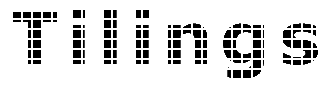#Penrose tilings of the plane

In 1973 Roger Penrose found a set of six tiles that force aperiodicity. Later, in 1974 he was able to reduce the set to two tiles. The shape of a pair of Penrose tiles can vary but the most common are the kite and the dart. These tiles are derived from a rhombus with angles 72 and 108 degrees.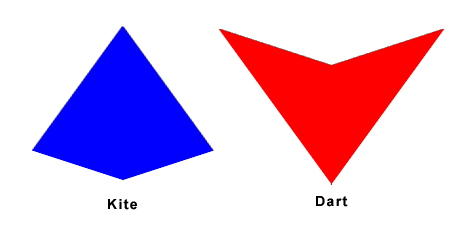Kite and Dart

There are different matching rules that enforce a way of joining the tiles in such a way as to create an aperiodic tiling. One of them consists of using two colors to label the corners and forcing fitting edges's corners to be of the same color. Another rule consists of drawing circular arcs of two colors on each side and edges must join arcs of the same color in order to join.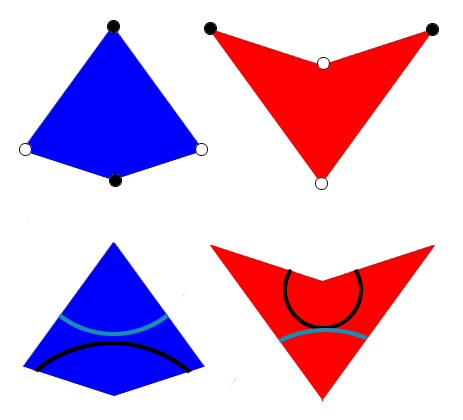Matching rules for the kite and dart

Another set of tiles can be derived by bisecting the kite and dart into isosceles triangles and merging the triangles into thick and thin rhombs.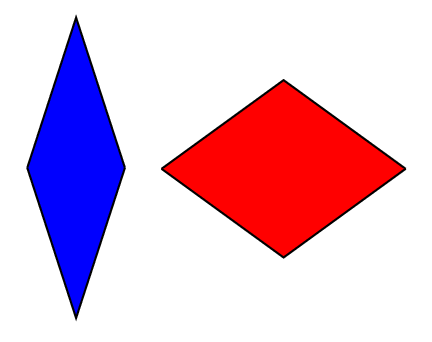Penrose rhombs

For example, a portion of a Penrose tile using the rhombs looks like this: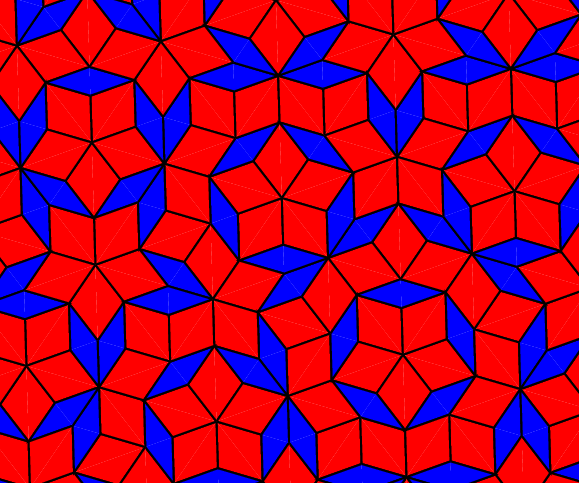Penrose tiling

#Generation of Penrose tilings

There are many ways of generating a Penrose tiling. The most obvious one is to make many copies of the tiles and start arranging them according to the matching rules. Using this approach one might reach a state where no more matchings are possible and we need to backtrack to make different choices for the position of the tiles.

Penrose tilings can also be generated using a substitution method. On every tile we can draw smaller generations of the tiles and similarly from smaller tiles we can generate larger tiles. This also means that there is a infinite number of Penrose tilings because we can continue substituting any number of times. The penrose tiling above was generated using this method with the postcript Penrose tiler described later.

A more involved method which lets us generate a Penrose tiling and to look at any portion of it consists of projecting a portion of a 5-dimensional lattice onto a 2-dimensional plane. By translating the plane we can draw any part of the Penrose tiling.

#Postscript Penrose tiling generator

The Postscript Penrose tiling generator can be found here: penrose.ps

This postscript program uses the substitution method to draw Penrose tilings. It uses a set of 4 tiriangular tiles that are generated by bisecting the thin rhomb along its short diagonal and the thick rhomb along its long diagonal. We will call these triangles TL, TR, tL and tR as shown below. The arrows on the sides specify the matching rules.Triangular tiles

Each of these triangles can be substituted by smaller triangles. The substitutions are: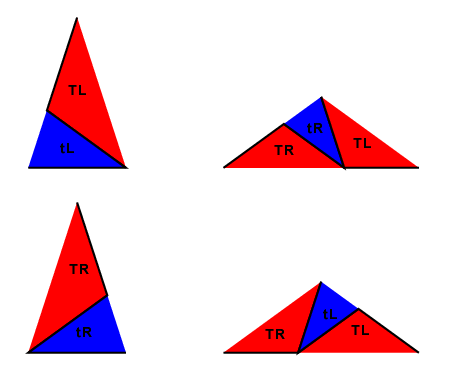Triangle substitutions

The program starts with a set of triangles and performs a substitution on each of the triangles. It does the same for the new tiling and so on for a specified amount of times. By using different starting sets and varying the number of iterations it can generate a large set of tilings, only limited by the postscript interpreter's stack size.

The next image shows the tilings generated starting with one TL and one TR triangle and different number of iterations.Multiple iterations

To set the starting triangle set open the penrose.ps file and change the value of the triangles variable. The value is an array of triangles and each triangle has the following format:
[type direction base]
type is 0 if it is a T triangle or 1 if it is a t triangle
direction if 0 for an L triangle or 1 for an R triangle
base consists of two arrays that represent the endpoints of the triangle's base: [x1 y1] [x2 y2].

For example, the value for triangles used in the image above was:
[[0 0 [3 8] [5 5]] [0 1 [5 5] [3 8]]]

The value of the variable N controls the number of iterations the program will perform.

Previous
| Index | Next